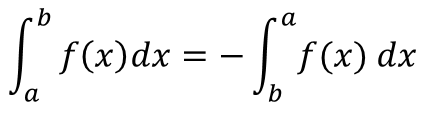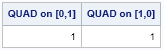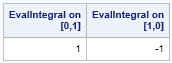In SAS software, you can use the QUAD subroutine in the SAS/IML language to evaluate definite integrals on an interval [a, b]. The integral is properly defined only for a < b, but mathematicians define the following convention, which enables you to make sense of reversing the limits of integration:Last week I was investigating a question that a SAS customer had posed to SAS Technical Support. In answering the question, it became important to know how the QUAD subroutine behaves if the left limit of integration is greater than the right limit. The QUAD subroutine supports an argument that specifies the limits of integration. The documentation states:

The simplest form of [the argument]provides the limits of the integration on one interval. The first element... should contain the left limit. The second element should be the right limit.... The elements of the vector must be sorted in an ascending order.

That's pretty explicit, so I thought I might get an error message if I specify a left limit that is greater than the right limit. However, that is not what happens! The following program shows the result of integrating the function f(x) = 4 x3 on the interval [0 1]. In the first call, I specify the limits of integration in ascending order. In the second call, I reverse the limits:

```proc iml; start Integrand(y); return( 4*y##3 ); finish;   call quad(w1, "Integrand", {0 1}); /* integral on [0 1] equals 1 */ call quad(w2, "Integrand", {1 0}); /* reverse limits */ print w1[L="QUAD on [0,1]"] /* should get 1 */ w2[L="QUAD on [1,0]"]; /* what do we get here? */```The QUAD subroutine does not give an error when the limits are in descending order. Instead, the routine silently sorts the limits and correctly evaluates the integral on the interval [0 1].

This seems like reasonable behavior, but for the problem that I was working on last week, I wanted to define a function where reversing the limits of integration results in reversing the sign of the integral. Now that I understood how the QUAD subroutine behaves, it was easy to write the function:

```/* Compute the integral on an interval where the lower limit of integration is a and the upper limit is b. If a <= b, return the integral on [a,b]. If a > b, return the opposite of the integral on [b,a] */ start EvalIntegral(FuncName, Limits); call quad(w, FuncName, Limits); a = Limits; b = Limits; return( choose(a<=b, w, -w) ); /* trick to handle a>b */ finish;   w3 = EvalIntegral("Integrand", {0 1} ); w4 = EvalIntegral("Integrand", {1 0} ); print w3[L="EvalIntegral on [0,1]"] w4[L="EvalIntegral on [1,0]"];```The EvalIntegral function is very simple. It evaluates the integral with the specified limits. If the limits of integration are in the usual (ascending) order, it returns the value of the integral. If the left limit of integration is greater than the right limit, it returns the opposite of the value that was computed. In other words, it implements the mathematical convention for reversing the limits of integration.

Reversing the limits of integration is a theoretical tool that rarely comes up in practice. However, if you ever need to compute a definite integral where the limits of integration are reversed, this blog post shows how to construct a function that obeys the mathematical convention.

ShareDistinguished Researcher in Computational Statistics

Rick Wicklin, PhD, is a distinguished researcher in computational statistics at SAS and is a principal developer of SAS/IML software. His areas of expertise include computational statistics, simulation, statistical graphics, and modern methods in statistical data analysis. Rick is author of the books Statistical Programming with SAS/IML Software and Simulating Data with SAS.

1.•